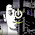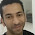## Monday, December 2, 2013

### Using The CCM Memory on the STM32

The STM32 series have non-contiguous memories divided into blocks, for example the STM32F4, has 2 (contiguous) blocks of SRAM connected to the bus matrix with different interconnects, and a Core Coupled Memory (CCM) block which is connected directly to the core.

This tight coupling of the CCM memory to the core, leads to zero wait states, in other words, the core has exclusive access to this memory block, so for example, while other bus masters are using the main SRAM the core can access the CCM. Therefore, the CCM block is commonly used for the stack and other critical OS data, this partitioning, allows the core to continue executing code while for example, a DMA transfer takes place. However, the CCM could also be used as an extra memory block, doing so is easy, and there are a few examples out there that show how, simply defining a section in the linker script will do:
```.ccm : {
. = ALIGN(4);
_sccm = .;
*(.ccm)
. = ALIGN(4);
_eccm = .;
}>CCM
```

And a section attribute is used to allocate memory into that section :
```const int8_t my_array __attribute__ ((section (".ccm")))= {....};
```

However, what if you want to load initialized data into that section ? some look-up tables for example?  using that section is not enough, see, the linker script makes the distinction between the Load Memory Address  (LMA) where data is stored initially, and the Virtual Memory Address (VMA) where the data should be loaded at runtime, if the LMA is not specified explicitly, it becomes the same as VMA.

You can see here that GDB loads the .ccm data into the CCM block (LMA=VMA=0x10000000) directly, while all other sections are loaded into the flash region (0x8xxxxxx):

```Loading section .ccm, size 0x4ebc lma 0x10000000
Loading section .isr_vector, size 0x188 lma 0x8000000
Loading section .text, size 0x9744 lma 0x8000188
Loading section .ARM, size 0x8 lma 0x80098cc
Loading section .init_array, size 0x8 lma 0x80098d4
Loading section .fini_array, size 0x4 lma 0x80098dc
Loading section .data, size 0xa30 lma 0x80098e0
Loading section .jcr, size 0x4 lma 0x800a310
```

While this may sound right, it's not, if GDB loads the .ccm section is loaded into SRAM directly, it will disappear after a power cycle! So instead, we want the LMA to be somewhere in the FLASH region (0x8xxxxxxx) and the VMA to be (0x10000000):
```_eidata = (_sidata + SIZEOF(.data) + SIZEOF(.jcr));
.ccm : AT ( _sidata + SIZEOF(.data) + SIZEOF(.jcr))
{
. = ALIGN(4);
_sccm = .;
*(.ccm)
. = ALIGN(4);
_eccm = .;
}>CCM
```

Note the .jcr is included in by some startup code for something related to Java, without adding the SIZEOF(.jcr) the .ccm will overlap that section, also note the _eidata symbol which will be referenced later in code. Now, when you try to load the elf, GDB prints:

```Loading section .isr_vector, size 0x188 lma 0x8000000
Loading section .text, size 0x9794 lma 0x8000188
Loading section .ARM, size 0x8 lma 0x800991c
Loading section .init_array, size 0x8 lma 0x8009924
Loading section .fini_array, size 0x4 lma 0x800992c
Loading section .data, size 0xa30 lma 0x8009930
Loading section .jcr, size 0x4 lma 0x800a360
Loading section .ccm, size 0x4ebc lma 0x800a364
```

Great, now the .ccm data is loaded into the FLASH region, we just need something to load it from FLASH to CCM in runtime, if you look at the startup code, there's an assembly function that copies initialized data from the flash to where it should be loaded in SRAM (the VMA), you need to do the same for the .ccm data, by either modifying the startup code, or perferrably, copying the data with a C function, so here it is:
```void load_ccm_section () __attribute__ ((section (".init")));
void load_ccm_section (){
extern char _eidata, _sccm, _eccm;

char *src = &_eidata;
char *dst = &_sccm;
while (dst < &_eccm) {
*dst++ = *src++;
}
}
```
Note that the function is placed into the .init section so it executes before main. Now in runtime, this function will load the data from FLASH into SRAM using the pointer defined in the linker script.

#### 15 comments:

1.hi,
can you post another topic or update this one with information about what contiguous memory and CCM are? and why do you ned to use it? thank you

1.Hi, contiguous memory means a single block of memory, no gaps in the address space, the STM32F4 has 3 blocks, two contiguous blocks (128KB total) and a separate CCM block (64KB), so you can't allocate a single array that spans the 3 blocks.
As for the CCM, I think I've talked about it enough in the post, but anyway, it's connected directly to the core on a separate bus, which means the core can access it while the main memory bus is used by say the DMA, you don't actually need to use it, but if you place the stack and critical system data there, the core should access them faster.

2.If I understand it correctly, it is the what you are doing:
1-you load the CCM data in Flash
2-when the microcontroller start it copy the data to CCM

Now I just don't understand if you are placing the stack into CCM or any other data.

Thank you :)

1.no stack just the data, I'm just using it as an extra memory block.

3.but on a faster way that using it on the FLASH, that's it?

1.I'm not sure I understand you, if you mean I can read the data from flash, yes, but that would be slow, I needed some arrays in memory, so I used the CCM as an extra block, there's some memory left there, so I may eventually move the stack there too.

4.Was what I meant, thank you for your patience :p

Just one more question about it, if we don't define the CCM as you did on the first code block above, what is the default use for CCM? the microcontroller doesn't use it at all or use ir like a normal RAM?

1.That depends on the linker script, most scripts either ignore it or just define a section for later use in runtime, but haven't seen any examples with initialized data, so I thought I'd post one, it just all depends on your application. and you're welcome, ask all you want.

5.Thank you for the NFO. It would be helpful if this guide showed how to put the stacks (main stack, process stack) into the CCM. I imagine that one needs to edit the linker script:
.stack :
{
. = ALIGN(8);
__stack_start = .;
PROVIDE(__stack_start = __stack_start);

. = ALIGN(8);
__main_stack_start = .;
PROVIDE(__main_stack_start = __main_stack_start);

. += __main_stack_size;

. = ALIGN(8);
__main_stack_end = .;
PROVIDE(__main_stack_end = __main_stack_end);

. = ALIGN(8);
__process_stack_start = .;
PROVIDE(__process_stack_start = __process_stack_start);

. += __process_stack_size;

. = ALIGN(8);
__process_stack_end = .;
PROVIDE(__process_stack_end = __process_stack_end);

. = ALIGN(8);
__stack_end = .;
PROVIDE(__stack_end = __stack_end);
} > ccm_ram AT > ccm_ram
If I want to combine the stack with some variables in the ccm, should I do anything else ?
Note that the CCM is not accessible by DMA.

1.if you're using malloc (linking with libc) you will need to fix _sbrk to check for heap/stack collision using the end of the heap (in main ram) not the stack, you could do that by using a different variable for the stack other than the one used by _sbrk (_estack) and if you need to place specific variables in the CCM you should use gcc's section attribute

I'm currently using the CCM for everything (stack,heap and data), check out my linker script:
https://github.com/iabdalkader/openmv/blob/master/src/stm32f4xx.ld

6.Also, please note, that the functions using CCM may run slower, because the D-Bus must be arbitrated with FLASH.
To utilize CCM w/o performance penalty, one can for example copy some IRQ handlers code and data into the CCM.

7.Really?
On STM32F405/407 (see Bus-Matrix above), the CCM is NOT connected to the I-Bus or S-Bus at all.
So, executing code from it might prove difficult...

8.Hi,

thanks for your example, but I ran into trouble. I tried to use your code to initialize a look up table in the CCM but all I get is a syntax error: nonconstant expression for load base

any clue?

9.Hy,
I read in the datasheets that the CCM Ram is supposed to be faster than the SRAM1 but when I run a little Test (10000x Incrementing a Variable in SRAM1 / CCMRAM, Variables were volatile and the Caches were disabled) I got the same results (both times 250us)????

1.I don't think so, they're both SRAMs. The CCM/TCM is just accessed exclusively. Note M4 doesn't have a cache.